# Test: Linear Algebra- 2

## 30 Questions MCQ Test GATE Computer Science Engineering(CSE) 2023 Mock Test Series | Test: Linear Algebra- 2

Description
Attempt Test: Linear Algebra- 2 | 30 questions in 90 minutes | Mock test for Computer Science Engineering (CSE) preparation | Free important questions MCQ to study GATE Computer Science Engineering(CSE) 2023 Mock Test Series for Computer Science Engineering (CSE) Exam | Download free PDF with solutions
QUESTION: 1

Solution:
QUESTION: 2

### How many of the following matrices have an eigenvalue 1?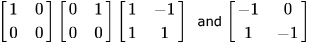Solution:

Characteristic equation is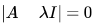(1)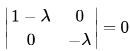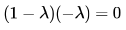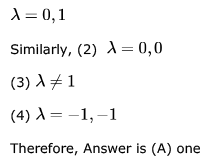*Multiple options can be correct
QUESTION: 3

### In questions 1.1 to 1.7 below, one or more of the alternatives are correct. Write the code letter(s) a, b, c, d corresponding to the correct alternative(s) in the answer book.  Marks will be given only if all the correct alternatives have been selected and no incorrect alternative is picked up. 1.1). The eigen vector (s) of the matrix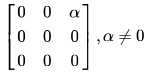is (are)

Solution:

Eigen values are: 0,0,0
The eigen vector should satisfy the equation: αz = 0

QUESTION: 4

What are the eigenvalues of the following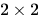matrix?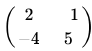Solution:

Let the eigen values be a,b
Sum of Eigen Values = Trace(Diagonal Sum)
⇒ a+b = 2+5 = 7
Product of Eigen Values = Det(A)
⇒ a.b = 6
Solving these we  get 1 and 6.. So, Option(B) is Correct ..

QUESTION: 5

Consider the following matrix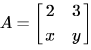If the eigenvalues of A are 4 and 8, then

Solution:

Sum of eighen values is equal to trace(sum of diagonal elements),and product of eighen values is equal to det of matrix
So 2+y=8+4 y =10
2y-3x=32
Solving this we get x =-4
Option d is ans

QUESTION: 6

a. Obtain the eigen values of the matrix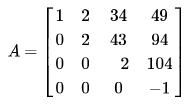Solution:

5(a) the eigen value for upper triangular/lower triangular/diagonal matrices are the diagonal elements of the matrix

QUESTION: 7

Consider the following determinant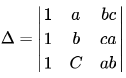Which of the following is a factor of Δ?

Solution:

R2->R2 - R1
R3 -> R3 - R2
you will gt det = (a-b)*(a-c)*(b+c)
in matrix operations, you cannot multiply rows or columns. That will not yield the same matrix. So abc is not correct

QUESTION: 8

Let A be a matrix such that Ak = 0. What is the inverse of I - A?

Solution:

Given AK = 0
I  -  Ak = I
I  -  Ak  = (I - A) (I + A + A2 + A3  +.........+Ak-1)
I  = (I - A) (I + A + A2 + A3  +.........+Ak-1)
(I - A)-1 =  (I + A + A2 + A3  +.........+Ak-1)

QUESTION: 9

F is an n*n real matrix. b is an n*1 real vector. Suppose there are two n*1 vectors, u and v such that, u ≠ v and Fu = b, Fv = b. Which one of the following statements is false?

Solution:

(A) : Correct. We are given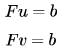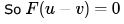Since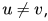so we have a non-zero solution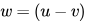to homogeneous equation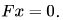Now any vector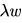is also a solution of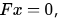and so we have infinitely many solutions ofand so determinant of F is zero.
(B) : Correct. Consider a vector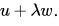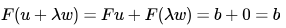So there are infinitely many vectors of the formwhich are solutions to equation Fx = b.
(C) : Correct. In option (a), we proved that vector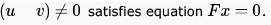(D) : False. This is not necessary.
So option (D) is the answer.

*Answer can only contain numeric values
QUESTION: 10

Perform the following operations on the matrix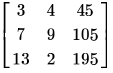i. Add the third row to the second row
ii. Subtract the third column from the first column.
The determinant of the resultant matrix is _____.

Solution:

Answer :- 0, because it is easy to see that first column and third column are multiple of each other.
Third column = First column * 15.
So rank is  < 3, so Determinant must be 0.
It stays zero as row & column transformations don't affect determinant.

*Answer can only contain numeric values
QUESTION: 11

In the LU decomposition of the matrix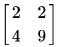, if the diagonal elements of U are both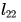, then the lower diagonal entry of L is_________________.

Solution:

Given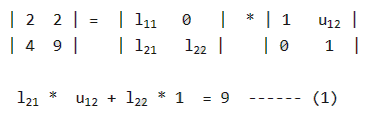We need to find l21 and u12 l21 *  1 + l22 * 0  = 4 l21 = 4

l11 * U12 + 0 * 1 = 2 l11 = 2 U12 = 1 Putting value of l21 and u12 in (1), we get 4 * 1 + l22 * 1 = 9 l22 = 5

QUESTION: 12

Let the characteristic equation of matrix  M be λ2 - λ - 1 = 0 . Then

Solution:

I think we can solve using Cayley- Hamilton Theorem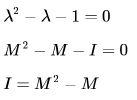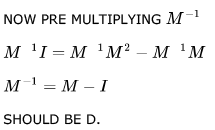QUESTION: 13

Consider the following statements:

• SI: The sum of two singularn n x n matrices may be non-singular
• S2: The sum of two n x n non-singular matrices may be singular

Which one of the following statements is correct?

Solution:

Yes A is correct option!, Both statements are True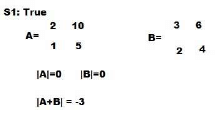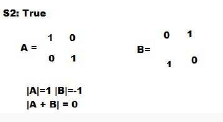QUESTION: 14

How many 4 x 4 matrices with entries from  have odd determinant?
Hint: Use modulo  arithmetic.

Solution:

whenever 1st row is 0 then its determent is 0 , and similarly if any 2 or more rows are linearly dependent then its |det|=0 In order to find the odd determinant the
1st row must be non zero --> totally(2^4-1) possibilities |0/1  0/1  0/1 0/1| like totally=16-1
2nd row must be non zero and not linearly depends on 1st row so--> totally (2^4-2) possibilities
for 3rd row it must be non-zero as well as not linearly depends on first 2 rows(not start with 0) --->totally (2^4-4)
for 4th row -->(2^4-8) :: total possibilities=(2^4-2^0) * (2^4-2^1) * (2^4-2^2) *(2^4-2^3)=15*14*12*8=20160 possible

QUESTION: 15

The rank of the matrix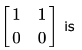Solution:

Rank of this matrix is 1 as the determint of 2nd order matrix is 0 and 1st order matrix is non zero so rank is 1

QUESTION: 16

Let A be an n × n matrix of the following form.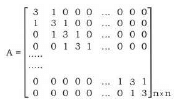What is the value of the determinant of A?

Solution:

Best part of this question  is dont solve by mathematical procedures. Verification is very easy in this question. just put n=1 , u ll get a matrix like .. find itdeterminant.. determinant = 3. now check options.
by putting n=1, i am getting following results..

A. 5
B. 7
C. 3
D. 3

now check for n=2.
determinantdeterminant = 9-1 =8
put n=2 in C,D.
C = 7
D = 8

QUESTION: 17

If matrix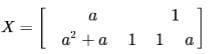and X2 - X + I = 0 (I is the identity matrix and  is the zero matrix), then the inverse of X is

Solution:

Given, X2 - X + I  = O
=>   X2 = X - I
=> X-1 (X2)  = X-1(X - I)    {Multiplying X-1 both sides..}
=> X = I - X-1
=> X-1 = I - X

Which gives Option (B)..

QUESTION: 18

The number of different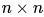symmetric matrices with each element being either 0 or 1 is: (Note: (2,X) is same as 2X)

Solution:

In symmetric matrix,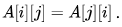So, we have choice only for either the upper triangular elements or the lower triangular elements. Number of such elements will be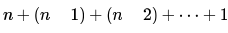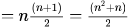Now, each element being either 0 or 1 means, we have 2 choices for each element and thus for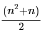elements we have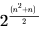possibilities.

QUESTION: 19

The rank of the following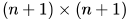matrix, where  is a real number is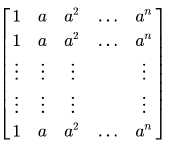Solution:

we can eliminate all other rows using row 1. in the last only 1 row will be left.

rank = no of non zero rows = 1

QUESTION: 20

The rank of the matrix given below is: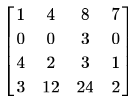Solution:
QUESTION: 21

Let A and B be real symmetric matrices of size. Then which one of the following is true?

Solution:

Given A = A' and B = B'
(AB)' = B'A' = BA

QUESTION: 22

The rank of matrix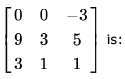Solution:

Determinant comes out to be 0. So, rank cannot be 3. The minor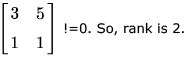QUESTION: 23

Let  A, B, C,D be  n * n  matrices, each with non-zero determinant. If ABCD = I, then B-1 is

Solution: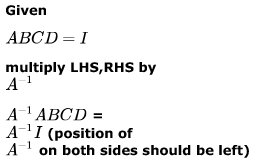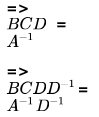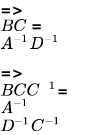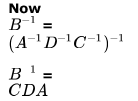QUESTION: 24

In an M × N  matrix all non-zero entries are covered in  rows and  columns. Then the maximum number of non-zero entries, such that no two are on the same row or column, is

Solution:

maximum number of non-zero entries, such that no two are on the same row or column

Any entry will be a member of some row and some column. So, with  rows we can have maximum  elements such that no row has a repeated element. Same is applicable for b columns also. So, combining both, answer should be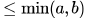.
We can also apply pigeonhole principle here. Let
P = min(a.b) be the number of holes. So, we can place up to
P non-zero entries (pigeons) and as soon as
(P + 1)th entry comes it must be making two entries in some column or row.

QUESTION: 25

The matrices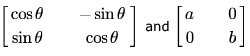commute under multiplication

Solution: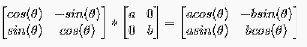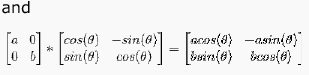The multiplication will commute if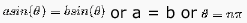QUESTION: 26

If M is a square matrix with a zero determinant, which of the following assertion (s) is (are) correct?
S1: Each row of M can be represented as a linear combination of the other rows
S2: Each column of M can be represented as a linear combination of the other columns
S3: MX = 0 has a nontrivial solution
S4: M has an inverse

Solution:

Since M has zero determinant, its rank is not full i.e. if M is of size 3*3, then its rank is not 3. So there is a linear combination of rows which evaluates to 0 i.e.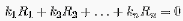and there is a linear combination of columns which evaluates to 0 i.e.Now any row Ri can be written as linear combination of other rows as :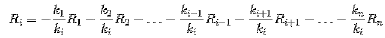Similar is the case for columns.
Now MX = 0 always has one solution : X = 0 (which is called trivial solution). Now if |M| = 0, then MX = 0 has non-trivial solutions also.
So (S1), (S2), and (S3) are true. So option D is correct.

QUESTION: 27

Consider the following system of linear equations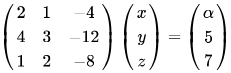Notice that the second and the third columns of the coefficient matrix are linearly dependent. For how many values of , does this system of equations have infinitely many solutions?

Solution:

Determinant=0. Therefore apply reduction method on (A|B)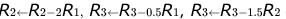obtain the resultant matrix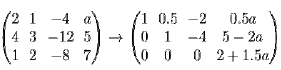or infinitely many solutions, we must have 2+1.5a=0 i.e., a=-4/3 so for only 1 value of a, this system has infinitely many solutions. So option (B) is correct.

QUESTION: 28

Consider the following system of linear equations :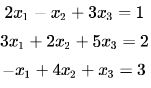The system of equations has

Solution:

rank of matrix = rank of augmented matrix = no of unknown = 3 so unique solution..

QUESTION: 29

How many solutions does the following system of linear equations have?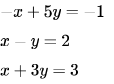Solution:

rank = r(A) = r(A|B) = 2
rank = total number of variables Hence, unique solution

QUESTION: 30

Consider the following set of equations x + 2y = 54 x+8y = 123x + 6y+3z = 15. This set

Solution:

There are no solutions.
If we multiply 1st equation by 4, we get
4x + 8y = 20
But 2nd equation says
4x + 8y = 12
Clearly, there can not be any pair of (x,y), which satisfies both equations.Use Code STAYHOME200 and get INR 200 additional OFF Use Coupon Code